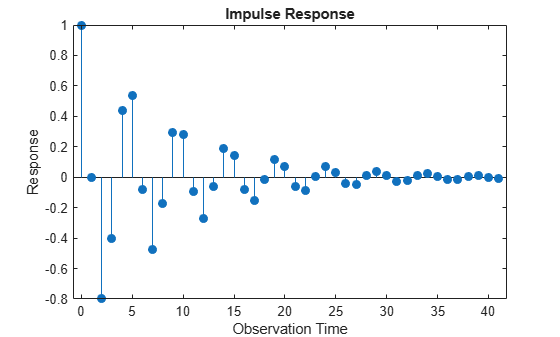# impulse

Generate regression model with ARIMA errors impulse response function (IRF)

## Syntax

``impulse(Mdl)``
``impulse(Mdl,numObs)``
``y = impulse(___)``

## Description

example

````impulse(Mdl)` plots a discrete stem plot of the impulse response function (IRF) for the regression model with ARIMA time series errors, `Mdl`, in the current figure window.```

example

````impulse(Mdl,numObs)` plots the IRF for `numObs` periods.```

example

````y = impulse(___)` returns the IRF in a column vector for any input argument combination in the previous syntaxes.```

## Examples

collapse all

Specify the following regression model with ARMA(2,1) errors:

`$\begin{array}{l}\begin{array}{c}{y}_{t}={X}_{t}\left[\begin{array}{c}0.1\\ -0.2\end{array}\right]+{u}_{t}\\ {u}_{t}=0.5{u}_{t-1}-0.8{u}_{t-2}+{\epsilon }_{t}-0.5{\epsilon }_{t-1},\end{array}\end{array}$`

where ${\epsilon }_{t}$ is Gaussian with variance 0.1.

```Mdl = regARIMA(Intercept=0,AR={0.5 -0.8},MA=-0.5, ... Beta=[0.1; -0.2],Variance=0.1);```

Time the calculation of and plot the impulse response function without specifying the number of observations.

`impulse(Mdl)`The model is stationary; the impulse response function decays in a sinusoidal pattern.

Specify the following regression model with ARMA(2,1) errors:

`$\begin{array}{l}\begin{array}{c}{y}_{t}={X}_{t}\left[\begin{array}{c}0.1\\ -0.2\end{array}\right]+{u}_{t}\\ {u}_{t}=0.5{u}_{t-1}-0.8{u}_{t-2}+{\epsilon }_{t}-0.5{\epsilon }_{t-1},\end{array}\end{array}$`

where ${\epsilon }_{t}$ is Gaussian with variance 0.1.

```Mdl = regARIMA(Intercept=0,AR={0.5 -0.8},MA=-0.5, ... Beta=[0.1; -0.2],Variance=0.1);```

Store the impulse response function for 15 periods.

`y = impulse(Mdl,15)`
```y = 15×1 1.0000 0 -0.8000 -0.4000 0.4400 0.5400 -0.0820 -0.4730 -0.1709 0.2930 ⋮ ```

The length of the output impulse response series is `numObs`. `y(1)` is the IRF at period 0, during which `impulse` shocks `Mdl`. `y(15)` is the IRF at period 14.

## Input Arguments

collapse all

Fully specified regression model with ARIMA errors, specified as a `regARIMA` model object created by `regARIMA` or `estimate`.

The properties of `Mdl` cannot contain `NaN` values.

Number of periods to include in the IRF (the number of periods for which `impulse` computes the IRF), specified as a positive integer.

If you specify `numObs`, `impulse` computes the IRF by filtering a unit impulse, followed by a vector of zeros of length `numObs` – 1, through the model `Mdl`. In this case, the filtering algorithm is efficient.

By default, `impulse` determines the number of periods in the IRF by using the polynomial division algorithm of the underlying lag operator polynomials. For more details, see Algorithms.

Example: `impulse(Mdl,10)` returns the IRF for 10 periods.

Data Types: `double`

## Output Arguments

collapse all

IRF, returned as a numeric column vector. If you specify `numObs`, `Y` has length `numObs`. If you do not specify `numObs`, the tolerances of the underlying lag operator polynomial division algorithm determine the length of `y`.

`y(j)` is the impulse response of yt at period `j` – 1. `y(0)` represents the impulse response during the period in which `impulse` applies the unit shock to the innovation (ε0 = 1).

collapse all

### Impulse Response Function

The impulse response function for regression models with ARIMA errors is the dynamic response of the system to a single impulse, or innovation shock, of unit size. The specific impulse response calculated by `impulse` is the dynamic multiplier, defined as the partial derivative of the output response with respect to an innovation shock at time 0.

For a regression model with ARIMA errors, yt, unconditional disturbances ut, and innovation series εt, the impulse response at time j, Ψj, is given by

`${\psi }_{j}=\frac{\partial {y}_{j}}{\partial {\epsilon }_{0}}=\frac{\partial {u}_{j}}{\partial {\epsilon }_{0}}.$`

Expressed as a function of time, the sequence of dynamic multipliers, Ψ1, Ψ2,..., measures the sensitivity of the process to a purely transitory change in the innovation process. `impulse` computes the impulse response function by shocking the system with a unit impulse ε0 = 1, with all past observations of yt and all future shocks of εt set to 0. The impulse response function is the partial derivative of the ARIMA process with respect to an innovation shock at time 0. Because of this, the presence of an intercept or a regression component corresponding to predictors in the model has no effect on the output.

This impulse response is sometimes called the forecast error impulse response, because the innovations, εt, can be interpreted as the one-step-ahead forecast errors.

## Tips

• To improve performance of the filtering algorithm, specify the number of observations, `numObs`, to include in the impulse response.

## Algorithms

• If you specify the number of periods `numObs`, `impulse` computes the IRF by filtering a unit shock followed by an appropriate length vector of zeros. The filtering algorithm is very fast and results in an IRF of `numObs` length.

• If you do not specify `numObs`, `impulse` implements this procedure:

1. Convert the error model to a pure moving average (MA) polynomial by using the relatively slow lag operator polynomial division algorithm.

2. Truncate the pure MA polynomial according to default tolerances because the polynomial is generally of infinite degree.

This procedure produces an IRF of generally unknown length. For more details, see `LagOp` and `mldivide`.

 Box, George E. P., Gwilym M. Jenkins, and Gregory C. Reinsel. Time Series Analysis: Forecasting and Control. 3rd ed. Englewood Cliffs, NJ: Prentice Hall, 1994.

 Enders, Walter. Applied Econometric Time Series. Hoboken, NJ: John Wiley & Sons, Inc., 1995.

 Hamilton, James D. Time Series Analysis. Princeton, NJ: Princeton University Press, 1994.

 Lütkepohl, Helmut. New Introduction to Multiple Time Series Analysis. New York, NY: Springer-Verlag, 2007.